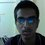# Motion

Can rectilinear motion be thought of as a circular motion with radius of curvature that tends to infinity.Note by Sai Prasanth Rao
6 years, 10 months ago

This discussion board is a place to discuss our Daily Challenges and the math and science related to those challenges. Explanations are more than just a solution — they should explain the steps and thinking strategies that you used to obtain the solution. Comments should further the discussion of math and science.

When posting on Brilliant:

• Use the emojis to react to an explanation, whether you're congratulating a job well done , or just really confused .
• Ask specific questions about the challenge or the steps in somebody's explanation. Well-posed questions can add a lot to the discussion, but posting "I don't understand!" doesn't help anyone.
• Try to contribute something new to the discussion, whether it is an extension, generalization or other idea related to the challenge.

MarkdownAppears as
*italics* or _italics_ italics
**bold** or __bold__ bold
- bulleted- list
• bulleted
• list
1. numbered2. list
1. numbered
2. list
Note: you must add a full line of space before and after lists for them to show up correctly
paragraph 1paragraph 2

paragraph 1

paragraph 2

[example link](https://brilliant.org)example link
> This is a quote
This is a quote
    # I indented these lines
# 4 spaces, and now they show
# up as a code block.

print "hello world"
# I indented these lines
# 4 spaces, and now they show
# up as a code block.

print "hello world"
MathAppears as
Remember to wrap math in $$ ... $$ or $ ... $ to ensure proper formatting.
2 \times 3 $2 \times 3$
2^{34} $2^{34}$
a_{i-1} $a_{i-1}$
\frac{2}{3} $\frac{2}{3}$
\sqrt{2} $\sqrt{2}$
\sum_{i=1}^3 $\sum_{i=1}^3$
\sin \theta $\sin \theta$
\boxed{123} $\boxed{123}$

Sort by:

Can $1$ be thought of as $0.99999999999999999.........................................$ ?

- 6 years, 10 months ago

yes if there are infinite number of 9's

- 6 years, 10 months ago

As far as i think rectilinear motion can not be considered as circular motion, because with an infinite radius and using the formula $v=\omega r$, even for a little bit of an angular velocity, our linear velocity should turn out be infinite which is not true, as it is possible to measure a speed of a moving car but not of infinity...

Moreover $0.9999999.....\infty$ tends to one, but considering it as one is an approximation, i.e. $0.99999....\infty \approx 1$, not an equality...:)

- 6 years, 10 months ago

When you say 0.99999.. with a large number of 9's it would tend to 1 but when you say infinite number of 9's it should be equal to 1 as it is an infinite G.P. As far as the note is concerned, a circle with infinite radius would give an object zero angular velocity. Another example-A polygon with infinite sides is a circle.

- 6 years, 10 months ago

You said it yourself...zero angular velocity and an infinite radius, how will you define their product i.e. Linear velocity, because you can't define $0\times \infty$

- 6 years, 10 months ago

however we can define the formula (m)(v^2)/r. As r is infinity the accelaration is zero. However how is it possible that in this way we get zero centripetal accelaration and in your method we get undefined centripetal accelaration.

- 6 years, 10 months ago

In my way, you don't get undefined centripetal acceleration, Although you get an undefined linear velocity. And moreover using $\frac { m{ v }^{ 2 } }{ r }$ , we see that an object moving in a circle must have a centripetal force. But, in this case, the centripetal force is zero, which clearly signifies that the object is not in circular motion

- 6 years, 10 months ago

yes we can conclude that the object is not in circular motion if we use the formula mv^2/r, but why can't we conclude the result while using the formula (mw^2)r

- 6 years, 10 months ago

Yes you can, as you can see 2 formulas of the same quantity are condradicting each other. That means that our earlier assumption that an object moving linearly is a part of a circle with an infinite radius is wrong...

- 6 years, 10 months ago

which standard are you in?

- 6 years, 10 months ago

11, is there anything wrong?

- 6 years, 10 months ago